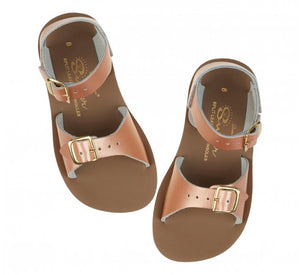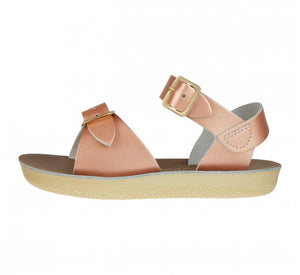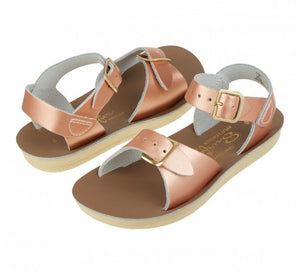# Salt Water Sandals / Sandalen / Surfer / Rose Gold

Regular price
€65.00
Regular price
€0.00
Sale price
€65.00
Unit price
per
Availability
Sold out

The Surfer is your ultimate two strap Jesus sandal. The adjustable double buckle sandal is the best all-rounder for both narrow and wide feet. The entire Sun-San Sandal range is defined by its 100% waterproof leather uppers, spongy urethane soft sole and rust proof buckles. They can be worn in water and handwashed with a gentle detergent. The Surfer red sandal is one of our best sellers, it is bright, fun and the perfect sandal for both boys and girls.
• 5 = 20/21 = 13 cm
• 6 = 22 = 14 cm
• 7 = 23 = 14,5 cm
• 8 = 24 = 15,5 cm
• 9 = 25 = 16,5 cm
• 10 = 26/27 = 17 cm
• 11 = 28 = 18 cm
• 12 = 29 = 19 cm
• 13 = 30/31 = 19,5 cm
• 1 = 32 = 20,5 cm
• 2 = 33 = 21,5 cm
• 3 = 34 = 22 cm

More Salt Water Sandals